# DP vs. Greedy (the activity-selection problem)

Updated:

⚠️ This post was created when I was in high school and is no longer maintained.

Algorithms for optimization problems typically go through a sequence of steps, with a set of choices at each step. For many optimization problems, using dynamic programming to determine the best choices is overkill; simpler, more efficient algorithms will do. A greedy algorithm always makes the choice that looks best at the moment. That is, it makes a locally optimal choice in the hope that this choice will lead to a globally optimal solution. —— CRLS

Whenever we don’t know what is the best, take a BRAVE, CLEVER guess. When I say brave, I mean guess them ALL, and by clever, I mean start guessing from a known or trivial base case (bottom-up). This is my understanding of what dynamic programming is. If we know what is the best choice, then be greedy and go for it 🏃

## 1. Problem

We have a set of activity in which each of them has a starting time $s_i$ and a finish time $f_i$. We assume that the activities are sorted in monotonically increasing order of finish time: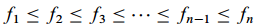For example,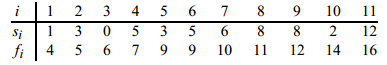In the above example, the optimal solution would be either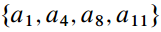or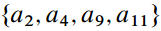## 2. Greed VS. DP

### 2.1 DP perspective

First off, let’s verify the optimal substructure of this problem.

We denote by $S_{ij}$ the set of activities that start after activity $a_i$ finishes and that finish before activity $a_j$ starts.

Suppose that we wish to find a maximum set of mutually compatible activities in $S_{ij}$, and suppose further that such a maximum set is $A_{ij}$, which includes some activity $a_k$.

By including $a_k$ in an optimal solution, we are left with two subproblems:

1. finding mutually compatible activities in the set $S_{ik}$ (activities that start after activity $a_i$ finishes and that finish before activity $a_k$ starts)
2. finding mutually compatible activities in the set $S_{kj}$

Let $A_{ik} = A_{ij} ∩ S_{ik}$ and $A_{kj} = A_{ij} ∩ S_{kj}$ then we will have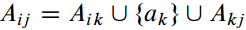In other words, the optimal, maximum-size set $A_{ij}$ consists of $\mid A_{ij} \mid = \mid A_{ik} \mid + 1 + \mid A_{kj} \mid$ mutaul compatible activities.

By this way (and “the usual cut-and-paste argument”), we characterised the optimal substructure which illustrates we can solve this problem by dynamic programming.

If we denote the size of an optimal solution for the set $S_{ij}$ by $c[i, j]$, then we would have the recurrence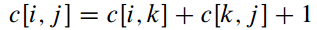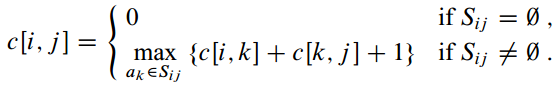Now, from the DP perspective, we can either develop a recursive algorithm and memoize it, or we could work bottom-up and fill in table entries as we go along.

### 2.2 Straightforward greedy strategy

Other than making an optimal decision after first solving all subproblems (build the DP table), for this problem, we can only consider one choice: the greedy one.

In this case, by greedy choice, I mean always choose the activity that has the earliest finish time, since that would leave the resource available for as many of the activities that follow it as possible.

Tips: This is not the only way to thinking of a greedy choice for this problem. In CLRS, Exercise 16.1-3 explores (and eliminates) other possibilities. Here is an interesting answer.

Unlike the DP mindset where two subproblems are considered, if we make the greedy choice, we have only one remaining subproblem to solve:

[ Find the activities that start after $a_1$ finishes with the earliest starting time. ]

(This is also the optimal choice)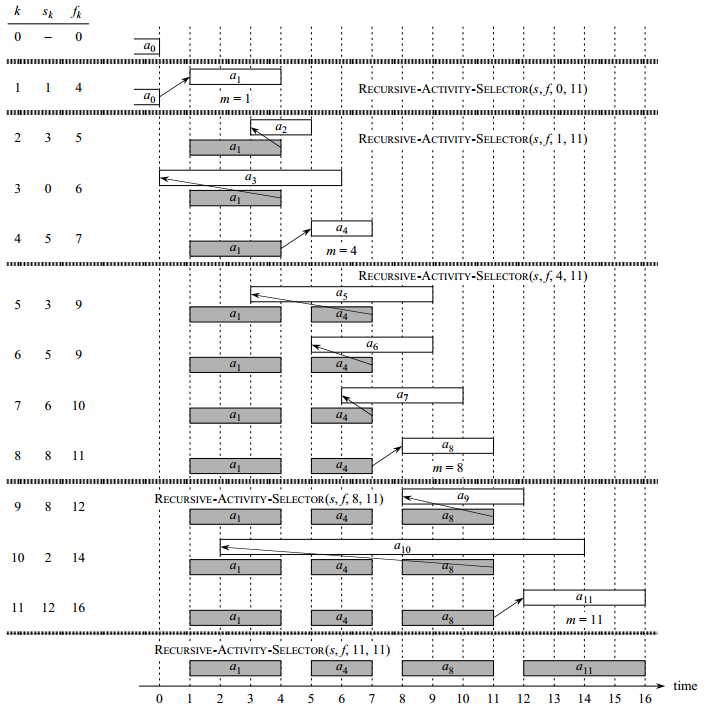Because no activity can finish at or before $s_1$. Thus, all compatible activities have to start after $f_1$.

The theorem blow shows that our intuition tells us the truth: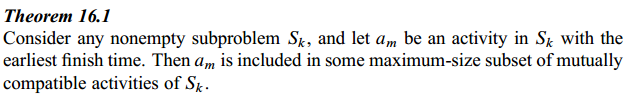To sum up:

• Instead of building a DP table, we can repeatedly choose the activity that finishes first, keep only the activities compatible with this activity, and repeat until no activities remain.

• We do not need to work bottom-up either, like a table-based DP algorithm;

• We start from a non-empty set that already contains the activity $a_1$ which has the earliest starting time;

• This algorithm only gives one of the answers that starts from the $a_1$ activity;

Greedy algorithms typically have this top-down design: make a choice and then solve a subproblem, rather than the bottom-up technique of solving subproblems before making a choice. —— CLRS

## 3. Pseudocode

### 3.1 Recursive version

-- Initial call (with fictious activity of index 0) --
Algorithm ActivitySelectorRecur(startTimeSet, finishTimeSet, 0, n);

Algorithm ActivitySelectorRecur(startTimeSet,
finishTimeSet,
prevActIndex, n):
nextActIndex := prevActIndex +1
-- check compatibility for betweeb the next and the previous activities --
While nextActIndex <= n and startTimeSet[nextActIndex] < finishTimeSet[prevActIndex] do
nextActIndex += 1

if nextActIndex <= n then
return activities[nextActIndex] + ActivitySelectorRecur(startTimeSet, finishTimeSet, nextActIndex, n))


### 3.2 Iterative version

Algorithm GreedyActivitySelector(startTimeSet, finishTimeSet, n)
selectedActs := Set( activities )
prevActIndex := 1

for nextActIndex := 2 to n do
if startTimeSet[nextActIndex] >= finishTimeSet[prevActIndex] then
selectedActs += activities[nextActIndex]
prevActIndex := nextActIndex

return selectedActs


## 4. Time complexity

For both recursive and iterative implementation, the greedy algorithm schedules a set of $n$ activities in $Θ(n)$ time, assuming that the activities were already sorted initially by their finish times (If not, we can sort them into this order in $O(nlogn)$ time, breaking ties arbitrarily. ).

Tags: